### Home > CALC > Chapter 2 > Lesson 2.2.2 > Problem2-72

2-72.
1.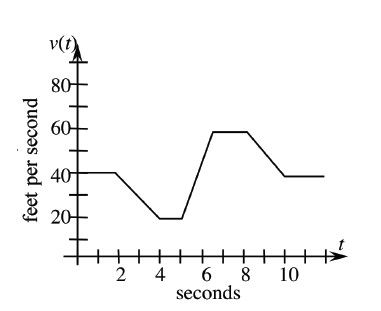THE CLUNKER
Tiffani has an old car that she is constantly repairing. One day, she was driving to school and started having problems with the car's fuel injection system. Her velocity (in feet per second) is shown below. Homework Help ✎

1. Recall that acceleration is the rate of change of velocity. How is acceleration represented on a velocity graph?

2. When was her acceleration negative?

3. What was her maximum acceleration?

4. Describe the motion of the vehicle when the acceleration was zero.

5. How far did she travel during the 12 seconds shown in the graph?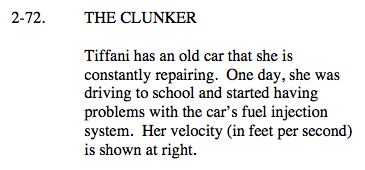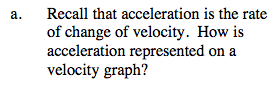$\text{acceleration }=\text{ rate of change of velocity }= \frac{\frac{\text{feet}}{\text{second}}}{\text{second}}.$

Notice what each axis represents.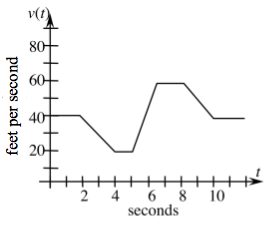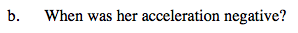negative acceleration = decreasing velocity. The first time this happens is between t = 2 and t = 4. When else does this happen?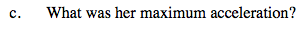On what interval is Tiffani's velocity increasing the most? Remember, this is a velocity graph.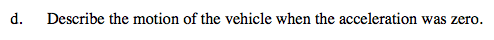0 acceleration means that velocity is not changing. It does NOT mean that velocity is 0. What does it mean then? Notice that Tiffani was moving with 0 acceleration during three intervals on her journey.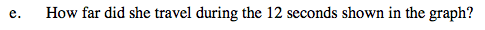Displacement can be determined by calculating the area under a velocity curve.